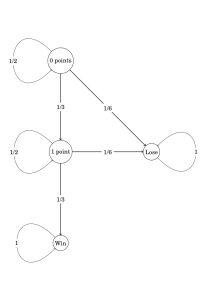I had a very limited time in which to finish these notes. Fortunately, I found the tkz-graph package, which made this a snap:
  1 2 3 4 5 6 7 8 9 10 11 12 13 14 15 16 17 18 19 20 21 22 23 24 25 26  \documentclass{standalone} \usepackage{tikz} \usepackage{fouriernc} \usepackage{tkz-graph} \begin{document} \begin{tikzpicture} \SetGraphUnit{5} \Vertex[x=0, y=10]{0 points}; \Vertex[x=0, y=5]{1 point}; \Vertex[x=0, y=0]{Win}; \Vertex[x=5, y=5]{Lose}; \Edge[style ={->}, label={$1/3$}]({0 points})({1 point}); \Edge[style ={->}, label={$1/3$}]({1 point})({Win}); \Edge[style ={->}, label={$1/6$}]({0 points})({Lose}); \Edge[style ={->}, label={$1/6$}]({1 point})({Lose}); \Loop[style ={->}, label={$1/2$}, labelstyle={fill=white}]({0 points}); \Loop[style ={->}, label={$1/2$}, labelstyle={fill=white}]({1 point}); \Loop[style ={->}, label={$1$}, dir=EA, labelstyle={fill=white}]({Lose}); \Loop[style ={->}, label={$1$}, labelstyle={fill=white}]({Win}); \end{tikzpicture} \end{document}
One slight issue I had was that the documentation for this package (at least on my computer, as retrieved by texdoc) was in French. Fortunately, I seem to have retained enough knowledge since I took the French language exam as a grad student that I could read most of the documentation.